• 斐波那契数列有两种常用的算法：递归算法和非递归算法。试分别分析两种算法的时间复杂度。   递归方式 递归方式代码： 递归结束条件可以不同，如果数列从第一个开始且为1，那么就是如下结束条件。 如果从第0个...

问题

来自王道考研数据结构书籍，思维拓展

斐波那契数列有两种常用的算法：递归算法和非递归算法。试分别分析两种算法的时间复杂度。

递归方式

递归方式代码：
递归结束条件可以不同，如果数列从第一个开始且为1，那么就是如下结束条件。
如果从第0个开始且第0个为0，那么结束条件就会改变：n等于0时返回0，n等于1时返回1

#include <stdio.h>
#include <stdlib.h>

int Fibonacci(int n){
if(n==1||n==2)
return 1;
else
return Fibonacci(n-1)+Fibonacci(n-2);
}

int main(int argc, char *argv[]) {
int n;
scanf("%d",&n);
int result = Fibonacci(n);
printf("%d",result);
return 0;
}

时间复杂度可通过下图分析：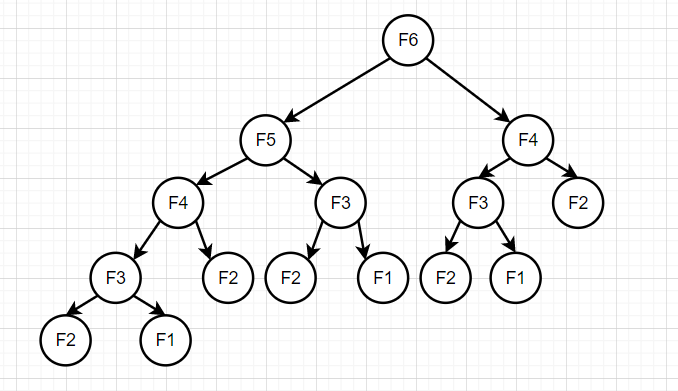如果是一个满二叉树的话，其时间复杂度就是O(2^n)。但实际上并不是满二叉树，所以比这个要小一点。网上有确切的值以及推导过程，大家可以看看。

非递归方式

代码如下：

#include<stdio.h>
#include<stdlib.h>

int Fibonacci(int n){
if(n<=2){
return 1;
}else{
int num1=1;
int num2=1;
int i;
for(i=2;i<n;i++){
num2=num1+num2;
num1=num2-num1;
}
return num2;
}
}

int main(){
int n;
scanf("%d",&n);
int result=Fibonacci(n);
printf("%d",result);
}

直接看for循环即可，语句重复执行的次数是n的数量级，所以时间复杂度为O(n)。

展开全文• 斐波那契数列——递归时间复杂度计算 画图：以F（6）为例：斐波那契数列到第六个数停止 每个节点运行都会开辟空间，使用时间 为了方便计算，把第五层的f（2）和f（1）放在第四层最右边，不会影响时间复杂度计算 ...

斐波那契数列——递归法时间复杂度计算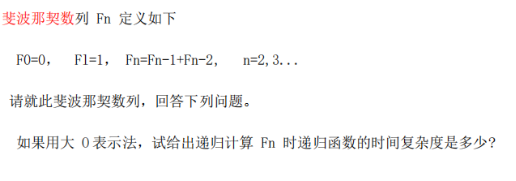画图：以F（6）为例：斐波那契数列到第六个数停止
每个节点运行都会开辟空间，使用时间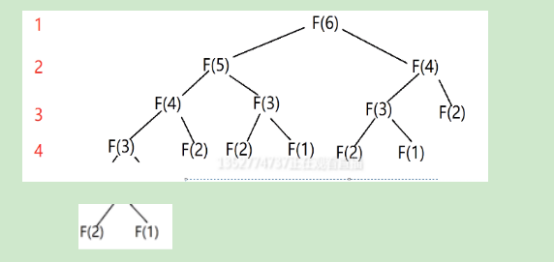为了方便计算，把第五层的f（2）和f（1）放在第四层最右边，不会影响时间复杂度计算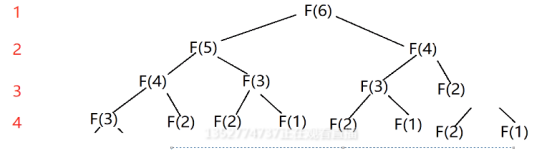第n层节点个数：2^n 个
前n层节点个数：1+2+4+……+2^n = (2 ^ n) - 1

F(6)有4层，推理：F（n） 有 n-2层
则F（n）一共有节点数 (2 ^ （n-2）) - 1 = ((2 ^n)/4)-1
计算时间复杂度规则：不看常数，不看系数，只看最高次数项
因此：O(F(n)) = O(2 ^n)

展开全文• JS递归代码实现 当参数为n时，时间复杂度为f(n) = f(n-1) + f(n-2) 当n为6时，树的高度为5即h=n-1的高度，共有15个节点即2^(h-1)-1个 时间复杂度为O(2^n) = f(2^n-1) - 1 空间复杂度为O(n) = f(n-1)

JS递归代码实现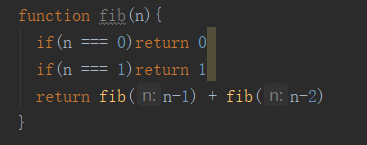当参数为n时，时间复杂度为f(n) = f(n-1) + f(n-2)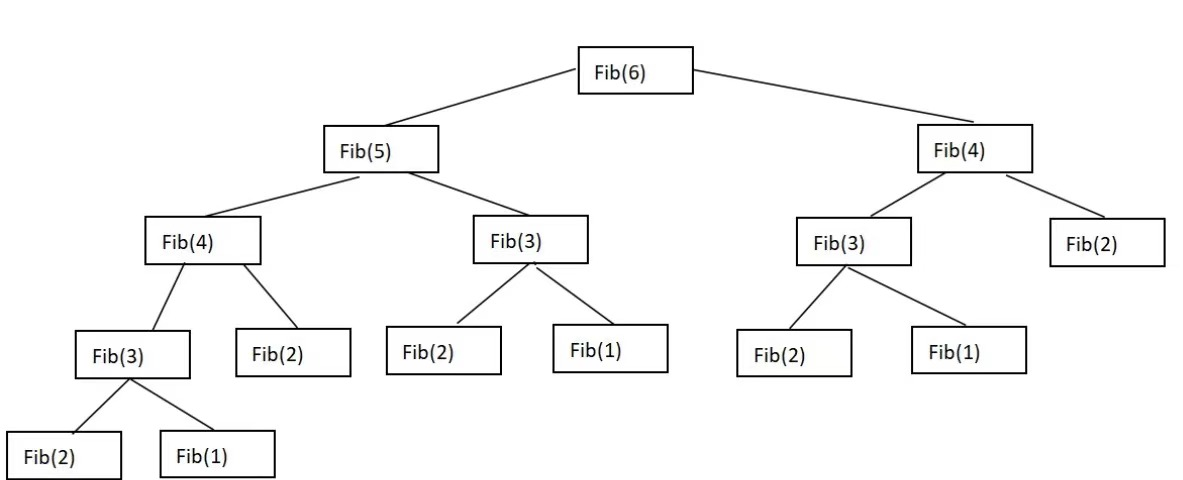当n为6时，树的高度为5即h=n-1的高度，共有15个节点即2^(h-1)-1个

时间复杂度为O(2^n) = f(2^n-1) - 1

空间复杂度为O(n) = f(n-1)

展开全文数据结构 算法 javascript
• 还跑了实验，但这是我人生中第 次看到有人犯将大数算术运算的复杂度视为 的“错误”了在一个更贴近现实的计算模型中，将两个 位大整数相加需要 的时间，而大整数相乘需要 (暴力算法)或 (FFT)的时间。pyt...

今天我在timeline上刷到了@Pika369 的这篇文章：https://zhuanlan.zhihu.com/p/165877869​zhuanlan.zhihu.com文章挺详细，还跑了实验，但这是我人生中第次看到有人犯将大数算术运算的复杂度视为的“错误”了

在一个更贴近现实的计算模型中，将两个位大整数相加需要的时间，而大整数相乘需要(暴力算法)或(FFT)的时间。python的乘法使用的是Karatsuba算法，下文简记为。可以看出，单纯按照“进行大整数运算的次数”来衡量算法复杂度是极不准确的，还需要考虑到大整数的长度以及算术运算的种类。知道了这点之后，我们可以重新分析一下原文中提到的各个计算Fibonacci数列算法的复杂度，并与实际运行时间进行对照

算法一：暴力递推

def F1(n):

a,b=1,1

for i in range(n-2): a,b=b,a+b

return b

根据fibonacci数的通项公式，我们可以看出第个fibonacci数的长度为。这里我们做了次大数加法，总复杂度为时运行时间为1.10s。把递归改成递推解决爆栈问题后跑的数据还是小看它了。

算法零：通项公式法

经评论区提醒补充分析一下这个，从数值计算的角度来说，根据数值分析的知识可知只需要对运算过程中涉及到的实数保留位精度就可以了。高精度实数开根可以在时间内完成，而exp可以用AGM方法在时间内完成从符号计算的角度来说，因为域扩张是一个有限扩张，所以维护下前的系数就可以了前的系数一定为0，不会产生精度问题(就是一个可怜的工具人)。实际运算和快速幂是等价的。

这里有用mpfr库写的C++代码，还是很快的，不过我们就不欺负python了。

算法二：矩阵的快速幂

import sympy as sym

def Matrix_fastPower(A,n):

size = A.shape

result = sym.eye(size)

base = A

power = n

while power > 0:

if power % 2 == 1:

result = result * base

power = power // 2

base = base * base

return result

def F2(n):

return Matrix_fastPower(sym.Matrix([[1,1],[1,0]]),n)[1,0]

把快速幂看成递归的话，递归深度为，大整数的算术运算总次数也为。但注意到递归过程中大整数的长度呈几何级数变化，即一次位大整数乘法的常数倍。时运行时间为81.6s，可以看出算法的常数比较大。

算法三：Fibonacci的一组恒等式。这个算法是Dijkstra在1978年发现的。

from functools import lru_cache

@lru_cache(None)

def F3(n):

if n == 1 or n == 2:

return 1

k = n // 2

a = F3(k)

b = F3(k + 1)

if n % 2:

return a * a + b * b

else:

return a * (2 * b - a)

复杂度的计算和算法二类似，因为递归过程中大整数的长度呈几何级数变化，总复杂度也为时运行时间为5.53s，常数比算法二小了很多。

注：原文代码在我本机的运行时间为5.71s，并且实际上“预先生成字典”对运行时间并没有什么影响。(与原作者确认过了)

算法3.1：

这里以对算法三的一个小改动为例，展示学会正确的复杂度分析可以如何帮助我们优化算法。

注意到算法三虽然进行了次大整数运算，但复杂度的瓶颈为最后一次乘法，这与实验结果是相符的：最后对的计算(根据的奇偶性需要1~2次乘法，这里为1次)花费了2.42s，占据了总运行时间的44%。可想而知，只要能在最后这次乘法上优化一点常数，总时间也可以被提升一个常数。这时候，我们需要一点关于凑因式分解的初中知识：

先考虑的情形。想法是多递归一层：令，则。令，直接用递归式计算的话这里需要一次长度为的乘法，以及三次长度为的乘法()。但我们可以换一种方法计算多项式的值：令，则。这样，长度为的乘法被减少到了两次(计算)。

其他情况同理。对于对于对于def F4(n):

a=F3(n//4)

b=F3(n//4+1)

t=a*(2*b-a)

t1=b*(2*a+b)

if n%4==0:

return t*(t1*2-3*t)

elif n%4==1:

c=t1-t

return t*t+c*c

elif n%4==2:

return (t1-t)*(t1+t)

else:

c=t1-t

return c*c+t1*t1时运行时间为5.05s，仅为算法三的91%。

(时运行时间比为时为时为。)

最后可以用以上技巧重新实现算法三，将运行时间进一步降低到原来的88%。起到的效果和这篇文章14%(为偶数时)。

def F3_(n):

if n==0: return 0,1

a,b=F3_(n//2)

t=a*(2*b-a)

t1=b*(2*a+b)

if n%2==0: return t,t1-t

else: return t1-t,t1

def F4_(n):

a,b=F3_(n//4)

t=a*(2*b-a)

t1=b*(2*a+b)

if n%4==0: return t*(t1*2-3*t)

elif n%4==1: return t*t+(t1-t)**2

elif n%4==2: return (t1-t)*(t1+t)

else: return (t1-t)**2+t1*t1

注：写文章的时候我不小心用32位python跑的，所以所有运行时间都那么慢。改回64位之后所有瓶颈是乘法的代码都可以快4倍左右，就不改正文了。

参考

展开全文• 斐波那契数列：前两项是1，后面的每项是其前两项之和。比如：1 1 2 3 5 8 13… 递归实现： def Fab(n): if n==0 or n==1: return 1 # 递归：函数的自身调用 return Fab(n-1) + Fab(n-2) 递归算法时间复杂度为...
• 直观感知是 O(2^n)，更精确一些的是 O((5/3)^n)
• 斐波那契数列是这样一个数列：1,1,2,3,5,8,13,21,34,55,89,144,233,377,610,987,1597,2584,4181,6765… 表达式为： (注：斐波那契数列也可从n=0开始，对应的F(0)=0) ...//递归实现斐波那契数列 int fib1(...数据结构 算法
• 一.结论 时间复杂度：O(2^n) 空间复杂度：O(n) ...一般算法分析都是使用RAM计算模型，而递归算法主要的时间花销就在于函数调用，也就是下图（2）中的子程序调用以及 return 语句。 三.具体分析 ...算法
• 递归代码实现： int Fib(n){ if (n==1 || n==2) return 1; else return Fib(n-1) + Fib(n-2); } 时间复杂度为 O(2^n) -------------二叉树的深度为h = n-1,叶子节点最多为2^（h-1)个，即为调用次数 空间...算法 数据结构
• 1、在学习数据结构这门课的过程中，发现斐波那契数列的递归算法以及非递归算法，以及其时间复杂度分析是一个小难点。所以特别总结一下。 斐波那契数列的表达式： Fibonacci数列简介： F(1)=1 F(2)=1 F(n)=F(n-...
• 关于斐波那契数列的简介：斐波那契数列，又称黄金分割数列，指的是这样一个数列：0、1、1、2、3、5、8、13、21、34、……在数学上，斐波纳契数列以如下被以递归的方法定义：F(0)=0，F(1)=1，F(n)=F(n-1)+F(n-2)(n≥2...
• 1.定义及递推公式斐波那契数列(Fibonacci sequence),又称黄金分割数列，因...1,1,2,3,5,8,13…即：f(1) = 1,f(2) = 1,f(3) = 2,f(4) = 3…添加0项后,Fibonacci数列归纳如下：f(n) = f(n-1) + f(n-2), n >= 2;f(...
• 我看在家修炼编程技术是不错的选择，「用Python实现斐波那契数列」是我们在知识星球中每周给大家安排的一道题，你也可以先思考一下有哪些实现方法，说不定哪天面试就能派上用场，终有一日当上CTO...
• 斐波拉契数列实现时间复杂度的分析算是一个难点，在考研数据结构中也经常会碰到，今天我们就来仔细分析下和解决掉这个问题。 1. 首先，我们先来看看递归形式斐波拉契数列的C语言实现： # include<stdio.h>...c语言 数据结构 算法
• 一、斐波那契数列的定义 二 、递归实现 三、循环实现 四、补充 一、斐波那契数列的定义 二 、递归实现 经典例题（杭电2041）: AC代码： #include <iostream> using namespace std; int f; ...C 算法 复杂度 斐波那契数列
• 首先了解线性递推数列的特征方程 （1）数列的特征方程： 假设一个数列：Xn+2 = C1Xn+1 + C2Xn 设有r,s使Xn+2 - rXn+1 = S(Xn+2-rXn); 所以Xn+2 = (s+r)Xn+1 - ...（2）使用二阶递推求斐波那契数列。   斐...
• 参考链接 https://www.cnblogs.com/zlshtml/p/11267310.html 非递归 class Solution { public: int fib(int N) { int n1=0,n2=1,n3; for(int i=2;i<=N;i++){ n3=n1+n2; n1=n2; n2=n3; } return n3;leetcode c++
• 这样一段代码的时间复杂度是多少呢？你可以先试着分析一下，然后再来看，我是怎么利用递归树来分析的。我们先把上面的递归代码画成递归树，就是下面这个样子： 这棵递归树的高度是多少呢？f(n) 分解为 f(n−1) 和 f...斐波那契数列 数据结构 时间复杂度
• 0. 问题描述在数学当中，由斐波那契数字(Fibonacci number，记作 )构成的序列，被称为斐波那契数列(Fibonacci sequence)。该数列中的每一个数字等于排在它前面的两个数字之和。数列从0和1开始： , 数列第n个(n>1)...
• 利用斐波那契数列测试递归及非递归算法的时间复杂度（工具：VS2015、C++，赠送精确计算耗时的类代码） 业余时间看了些关于时间复杂度的资料，就想着根据资料写个代码测试一下，本人尚属菜鸟，欢迎各位看官提出宝贵...递归 时间复杂度 c++ VS2015
• 斐波那契数列我写了四种实现方法： 第一种：递归实现 long long Fib(int n)//递归 { if (n ) { return n; } return Fib(n - 1) + Fib(n - 2); }时间复杂度：O(2^n) 空间复杂度：O(n) 第二种：非递归实现 ...递归 空间复杂度 C++
• java实现 class Fibonacci{ //递归 public static int fibonacci_recursion(int n){ if(n==0||n==1){ return 1; } if(n>=2){ return fibonacci_recursion(n-1)+fibonacci_recursion(n-2); } return数据结构
• 1.时间复杂度： 时间复杂度其实即使算法执行次数n的某个函数f(n)，进而分析f(n)随n的变化情况并确定T(n)的数量级。这里用"O"来表示数量级，时间复杂度的表达式为。 T(n)=O(f(n)); 它表示随着问题规模的...
• 5.斐波那契递归实现 6.怎么在时间复杂度O(1)，空间复杂度O(1)下计算斐波那契数 1.斐波那契数简介 斐波那契数列Fibonacci sequence），又称黄金分割数列、因数学家莱昂纳多·斐波那契（Leonardoda Fibonacci...
• 　斐波那契数列，又称黄金分割数列，指的是这样一个数列：0、1、1、2、3、5、8、13、21、34、……在数学上，斐波纳契数列以如下被以递归的方法定义：F（0）=0，F（1）=1，F（n）=F(n-1)+F(n-2)（n≥2，n∈N*）在现代...
• 斐波那契数列概述：斐波那契数列，又称黄金分割数列，指的是这样一个数列：0、1、1、2、3、5、8、13、21、34、……在数学上，斐波纳契数列以如下被以递归的方法定义：F(0)=0，F(1)=1，F(n)=F(n-1)+F(n-2)(n≥2，n∈N...
• 斐波那契数列算法及时间复杂度分析 斐波那契数列是一个很有意思的数列,应用领域非常广. 定义: F(n+1)=F(n)+F(n-1) 有意思的是,F(n)/F(n+1)趋于黄金分割0.618. 如何计算斐波那契数呢? 最朴素的思想,利用...斐波那契...